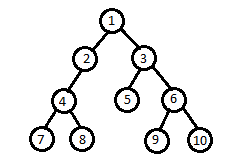Problem title
Difficulty
Avg time to solve

LCA of three Nodes
Easy
20 mins
Sum Of Infinite Array
Moderate
35 mins
Pair Sum
Easy
15 mins
Reverse The Array
Easy
15 mins
Sum or Product
Easy
15 mins
Two Sum
Easy
10 mins
Find Duplicate in Array
Easy
15 mins
Flower Shop
Easy
--
Sort 0 1 2
Easy
22 mins
Maximum Subarray Sum
Hard
35 mins3205

# LCA of three Nodes

Difficulty: EASY
Avg. time to solve
20 min
Success Rate
60%

Problem Statement

#### You have been given a Binary Tree of 'N' nodes where the nodes have integer values and three integers 'N1', 'N2', and 'N3'. Find the LCA(Lowest Common Ancestor) of the three nodes represented by the given three('N1', 'N2', 'N3') integer values in the Binary Tree.

##### For example:``````For the given binary tree: the LCA of (7,8,10) is 1
``````
##### Note:
``````All of the node values of the binary tree will be unique.

N1, N2, and N3  will always exist in the binary tree.
``````
##### Input Format:
``````The first line of input contains a single integer T, representing the number of test cases or queries to be run.

Then the T test cases follow.

The first line of each test case contains three single space-separated integers N1, N2, and N3, denoting the nodes of which LCA is to be calculated.

The second line of each test case contains elements in the level order form. The line consists of values of nodes separated by a single space. In case a node is null, we take -1 in its place.

For example, the input for the tree depicted in the below image would be :
````````````1
2 3
4 -1 5 6
-1 7 -1 -1 -1 -1
-1 -1
``````

#### Explanation :

``````Level 1 :
The root node of the tree is 1

Level 2 :
Left child of 1 = 2
Right child of 1 = 3

Level 3 :
Left child of 2 = 4
Right child of 2 = null (-1)
Left child of 3 = 5
Right child of 3 = 6

Level 4 :
Left child of 4 = null (-1)
Right child of 4 = 7
Left child of 5 = null (-1)
Right child of 5 = null (-1)
Left child of 6 = null (-1)
Right child of 6 = null (-1)

Level 5 :
Left child of 7 = null (-1)
Right child of 7 = null (-1)

The first not-null node (of the previous level) is treated as the parent of the first two nodes of the current level. The second not-null node (of the previous level) is treated as the parent node for the next two nodes of the current level and so on.
The input ends when all nodes at the last level are null (-1).
``````
##### Note :
``````The above format was just to provide clarity on how the input is formed for a given tree.
The sequence will be put together in a single line separated by a single space. Hence, for the above-depicted tree, the input will be given as:

1 2 3 4 -1 5 6 -1 7 -1 -1 -1 -1 -1 -1
``````
##### Output Format:
``````For each test case,  return the node representing LCA.
``````
##### Note
``````You do not need to print anything, it has already been taken care of. Just implement the given function.
``````
##### Constraints:
``````1 <= T <= 10
1 <= N <= 10^5
0 <= node data <= 10^9
0 <= N1 <= 10^9
0 <= N2 <= 10^9
0 <= N3 <= 10^9

Time Limit: 1sec
``````
##### Sample Input 1:
``````1
8 9 11
1 2 3 4 5 6 7 8 9 -1 -1 -1 -1 -1 -1 10 -1 11 -1 -1 -1 -1 -1
``````
##### Sample Output 1:
``````4
``````
##### Sample Input 2:
``````2
7 8 2
1 2 3 4 5 6 7 8 9 -1 -1 -1 -1 -1 -1 10 -1 11 -1 -1 -1 -1 -1
5 6 7
1 2 3 4 5 6 7 8 9 -1 -1 -1 -1 -1 -1 10 -1 11 -1 -1 -1 -1 -1
``````
##### Sample Output 2:
``````1
1
``````
##### Explanation to Sample Input 2:
``````For both inputs, the binary tree will be represented as
````````````For the first test case, the LCA of 7,8,2 in the given tree is 2

For the second test case. the LCA of 5,6,7 in the given tree is 1,
``````Console